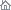Home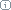About Us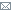ContactBlogWhat's NewProductsBuy NowDownloadsForumGeneXproTools Online Guide Learn how to use the 5 modeling platforms of GeneXproTools with the Online GuideLast update: February 19, 2014Category Mapping

GeneXproTools supports all kinds of categorical variables, both as part of entirely categorical datasets or intermixed with numerical variables. In both cases the categories in all categorical variables are automatically replaced by numerical values so that you can start modeling straightaway.

GeneXproTools uses simple heuristics to make this initial mapping, but then lets you choose more meaningful mappings in the Category Mapping Window.

Dependent categorical variables are also supported but they are handled differently in classification and logistic regression problems with more than two classes. In these cases the mapping is made so that only one class is singled out, resulting in a binomial outcome, such as {0, 1} or {-1, 1}, which can then be used to create classification or logistic regression models. The merging of the response variable in classification and logistic regression is handled in the Class Merging & Discretization Window.

In regression problems, dependent categorical variables are handled exactly as any other categorical variable, that is, the categories in the response variable are also converted to numerical values using user-defined mappings.

The beauty and power of GeneXproTools support for categorical variables goes beyond giving you access to a sophisticated and extremely useful tool for changing and experimenting with different mappings easily and quickly by trying out different scenarios and seeing immediately how they impact on modeling. Indeed GeneXproTools also generates code that supports data in exactly the same format that was loaded into GeneXproTools. This means that all the code generated both for external model deployment or for scoring internally in GeneXproTools, also supports categorical variables. Below is an example in C++ of a logistic regression model with 12 variables, 7 of which are categorical.
```
//------------------------------------------------------------------
// Logistic regression model generated by GeneXproTools 5.0 on 5/17/2013 4:13:11 PM
// GEP File: D:\GeneXproTools\Version5.0\OnlineGuide\LoanRisk_03a.gep
// Training Records:  667
// Validation Records:   333
// Fitness Function:  Bounded ROC, Logistic Threshold
// Training Fitness:  726.124343110239
// Training Accuracy: 76.76% (512)
// Validation Fitness:   777.537892479522
// Validation Accuracy:  79.58% (265)
//------------------------------------------------------------------

#include "math.h"
#include "string.h"

double gepModel(char* d_string[]);
double gep3Rt(double x);
void TransformCategoricalInputs(char* input[], double output[]);

double gepModel(char* d_string[])
{
const double G3C5 = -4.97848445081942;

double d;
TransformCategoricalInputs(d_string, d);

double dblTemp = 0.0;

dblTemp = (pow(d,4)+d);
dblTemp += exp(d);
dblTemp += (((d*pow(gep3Rt((d+G3C5)),3))-d)-d);
dblTemp += ((d*(d+d))-(((d*d)*d)*gep3Rt(d)));

const double SLOPE = 6.9596006314631E-03;
const double INTERCEPT = 3.45748287482188E-02;

double probabilityOne = 1.0 / (1.0 + exp(-(SLOPE * dblTemp + INTERCEPT)));
return probabilityOne;
}

double gep3Rt(double x)
{
return x < 0.0 ? -pow(-x,(1.0/3.0)) : pow(x,(1.0/3.0));
}

void TransformCategoricalInputs(char* input[], double output[])
{
if(strcmp("A11", input) == 0)
output = 1.0;
else if(strcmp("A12", input) == 0)
output = 2.0;
else if(strcmp("A13", input) == 0)
output = 3.0;
else if(strcmp("A14", input) == 0)
output = 4.0;
else output = 0.0;

output = atof(input);

if(strcmp("A30", input) == 0)
output = 1.0;
else if(strcmp("A31", input) == 0)
output = 2.0;
else if(strcmp("A32", input) == 0)
output = 3.0;
else if(strcmp("A33", input) == 0)
output = 4.0;
else if(strcmp("A34", input) == 0)
output = 5.0;
else output = 0.0;

if(strcmp("A61", input) == 0)
output = 1.0;
else if(strcmp("A62", input) == 0)
output = 2.0;
else if(strcmp("A63", input) == 0)
output = 3.0;
else if(strcmp("A64", input) == 0)
output = 4.0;
else if(strcmp("A65", input) == 0)
output = 5.0;
else output = 0.0;

output = atof(input);

if(strcmp("A91", input) == 0)
output = 1.0;
else if(strcmp("A92", input) == 0)
output = 2.0;
else if(strcmp("A93", input) == 0)
output = 3.0;
else if(strcmp("A94", input) == 0)
output = 4.0;
else output = 0.0;

if(strcmp("A101", input) == 0)
output = 1.0;
else if(strcmp("A102", input) == 0)
output = 2.0;
else if(strcmp("A103", input) == 0)
output = 3.0;
else output = 0.0;

output = atof(input);

if(strcmp("A121", input) == 0)
output = 1.0;
else if(strcmp("A122", input) == 0)
output = 2.0;
else if(strcmp("A123", input) == 0)
output = 3.0;
else if(strcmp("A124", input) == 0)
output = 4.0;
else output = 0.0;

output = atof(input);

output = atof(input);

if(strcmp("A191", input) == 0)
output = 1.0;
else if(strcmp("A192", input) == 0)
output = 2.0;
else output = 0.0;
}
```

Related Tutorials:

Related Videos:

 Leave Feedback Please enter the number below using the combo boxes before sending your feedback. 3 8 4 0 1 2 3 4 5 6 7 8 9   0 1 2 3 4 5 6 7 8 9   0 1 2 3 4 5 6 7 8 9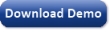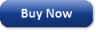Time Limited Trial

Released February 19, 2014

Last update: 5.0.3883New Entries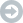Subscribe to the GEP-List

 3 8 4 0 1 2 3 4 5 6 7 8 9   0 1 2 3 4 5 6 7 8 9   0 1 2 3 4 5 6 7 8 9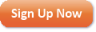Home | What's New | Products | Buy Now | Downloads | Quick Tour | Support | Contact Us | About Gepsoft | Sign UpForum | Blog | Videos | Tutorials | Server Knowledge Base | Logistic Regression Guide | Terms of Use | Privacy & Cookies